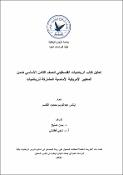## ANALYSIS OF THE PALESTINIAN MATHEMATICS BOOK FOR THE EIGHTH GRADE WITHIN THE COMMON CORE STATE STANDARDS FOR MATHEMATICS (CCSSM)2022-03-10
AL-qasim, Enas
##### Publisher
An-Najah National University
##### Abstract
The current study aimed to determine on the availability of content standards determined by the Common Core American State for Mathematics (CCSSM) in the five following content areas: numbers, algebra, functions, geometry and measurement, statistics and probability, in the content of the mathematics curriculum for the eighth grade. The researcher utilized the descriptive analytical approach. To achieve that, the researcher translated the Common Core American Standards for Mathematics (CCSSM) for the eighth grade, and then prepared a list of those standards that were exploited in preparing a content analysis card which formed the study tool. The tool was used as a reference in elaborating on the content of the Math curriculum which is the Palestinian eighth grade primary applied in the year 2021-2022 AD, with two books. After ensuring the validity and stability of the tool, as well as conducting the convenient statistical treatments, the results of the study showed the availability of the content standards set by the Common Core American State for Mathematics (CCSSM) in the three content areas, namely: Numbers Algebra, geometry and measurement, where the field of algebra ranked first with a percentage of (62.2%), and the field of numbers ranked second with a percentage of 19%. The field of engineering and measurement ranked third with a rate of (18.8%), while the content standards set by the Common Core American State for Mathematics (CCSSM) were not available in the areas of: functions, statistics and probability. According to the aforementioned results, the study recommended the need to concentrate on the indicators of unfulfilled standards in the developed mathematics textbook for the eighth grade, which were identified by (CCSSM). Moreover, it suggested to reconsider the weaknesses in the mathematics textbook for the eighth grade and to work to address them. There is a necessity to focus on strengths, to enrich the new edition of the mathematics book for the eighth grade, and to monitor paragraphs belonging to the areas (functions, statistics and probability) recognized by (CCSSM).
##### Description
The current study aimed to determine on the availability of content standards determined by the Common Core American State for Mathematics (CCSSM) in the five following content areas: numbers, algebra, functions, geometry and measurement, statistics and probability, in the content of the mathematics curriculum for the eighth grade. The researcher utilized the descriptive analytical approach. To achieve that, the researcher translated the Common Core American Standards for Mathematics (CCSSM) for the eighth grade, and then prepared a list of those standards that were exploited in preparing a content analysis card which formed the study tool. The tool was used as a reference in elaborating on the content of the Math curriculum which is the Palestinian eighth grade primary applied in the year 2021-2022 AD, with two books. After ensuring the validity and stability of the tool, as well as conducting the convenient statistical treatments, the results of the study showed the availability of the content standards set by the Common Core American State for Mathematics (CCSSM) in the three content areas, namely: Numbers Algebra, geometry and measurement, where the field of algebra ranked first with a percentage of (62.2%), and the field of numbers ranked second with a percentage of 19%. The field of engineering and measurement ranked third with a rate of (18.8%), while the content standards set by the Common Core American State for Mathematics (CCSSM) were not available in the areas of: functions, statistics and probability. According to the aforementioned results, the study recommended the need to concentrate on the indicators of unfulfilled standards in the developed mathematics textbook for the eighth grade, which were identified by (CCSSM). Moreover, it suggested to reconsider the weaknesses in the mathematics textbook for the eighth grade and to work to address them. There is a necessity to focus on strengths, to enrich the new edition of the mathematics book for the eighth grade, and to monitor paragraphs belonging to the areas (functions, statistics and probability) recognized by (CCSSM).
##### Keywords
Analysis book, Eighth grade, Comon Core State Standards for Mathematics (CCSSM).